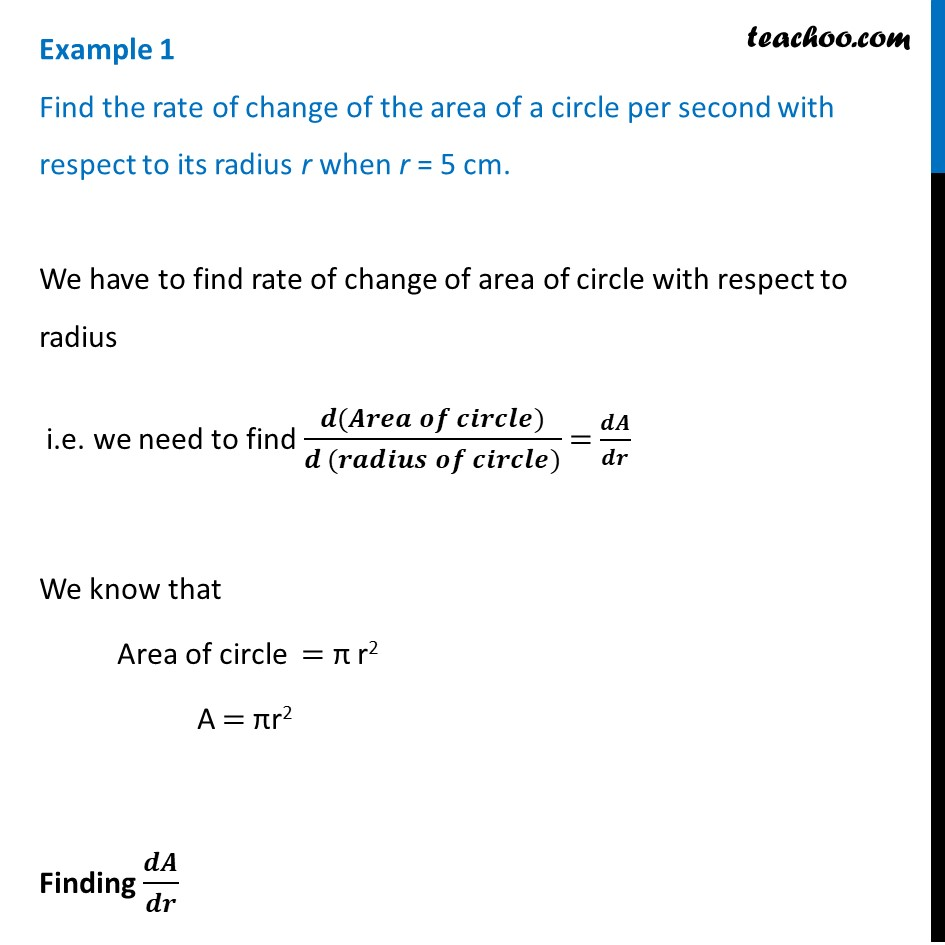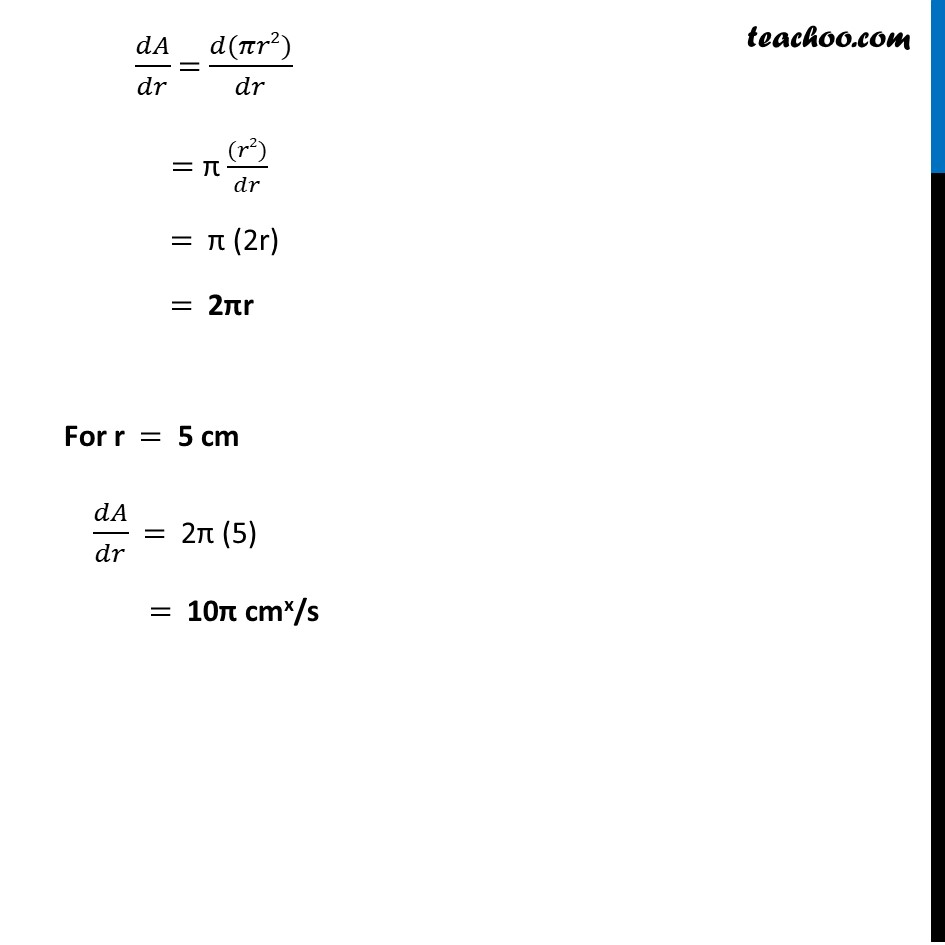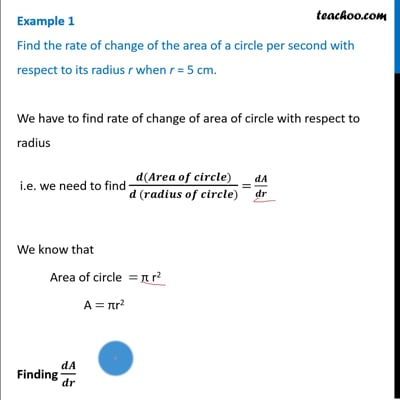Finding rate of change

Chapter 6 Class 12 Application of Derivatives
Concept wiseThis video is only available for Teachoo black users

Introducing your new favourite teacher - Teachoo Black, at only ₹83 per month

### Transcript

Example 1 Find the rate of change of the area of a circle per second with respect to its radius r when r = 5 cm.We have to find rate of change of area of circle with respect to radius i.e. we need to find (𝒅(𝑨𝒓𝒆𝒂 𝒐𝒇 𝒄𝒊𝒓𝒄𝒍𝒆))/(𝒅 (𝒓𝒂𝒅𝒊𝒖𝒔 𝒐𝒇 𝒄𝒊𝒓𝒄𝒍𝒆)) = 𝒅𝑨/𝒅𝒓 We know that Area of circle = π r2 A = πr2 Finding 𝒅𝑨/𝒅𝒓 𝑑𝐴/𝑑𝑟 = (𝑑(𝜋𝑟2))/𝑑𝑟 = π ((𝑟2))/𝑑𝑟 = π (2r) = 2πr For r = 5 cm 𝑑𝐴/𝑑𝑟 = 2π (5) = 10π cmx/s